# Visualizations

Databricks supports various types of visualizations out of the box.

All notebooks support DataFrame visualizations using the `display` function. The `display` function also supports rendering image data types and various machine learning visualizations.

Databricks programming language notebooks (Python, Scala, R) support HTML graphics using the `displayHTML` function; you can pass it any HTML, CSS, or JavaScript code. This function support interactive graphics using JavaScript libraries such as D3.

## `display` function

The easiest way to create a DataFrame visualization in Databricks is to call `display(<dataframe-name>)`. For example, if you have a Spark DataFrame `diamonds_df` of a diamonds dataset grouped by diamond color, computing the average price, and you call

```from pyspark.sql.functions import avg

display(diamonds_df.select("color","price").groupBy("color").agg(avg("price")))
```

A table of diamond color versus average price displays.Note

If you see `OK` with no rendering after calling the `display` function, mostly likely the DataFrame or collection you passed in is empty.

If you register a Spark DataFrame as a table, you can also query it with SQL to create SQL visualizations.

### pandas DataFrames

In Databricks Runtime 5.1 and above, `display` supports pandas DataFrames.

Note

If you call a pandas or koalas DataFrame without `display`, the table is rendered as it would be in Jupyter.

### Visualization types

Click the bar chart icon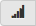to display a chart of tabular information: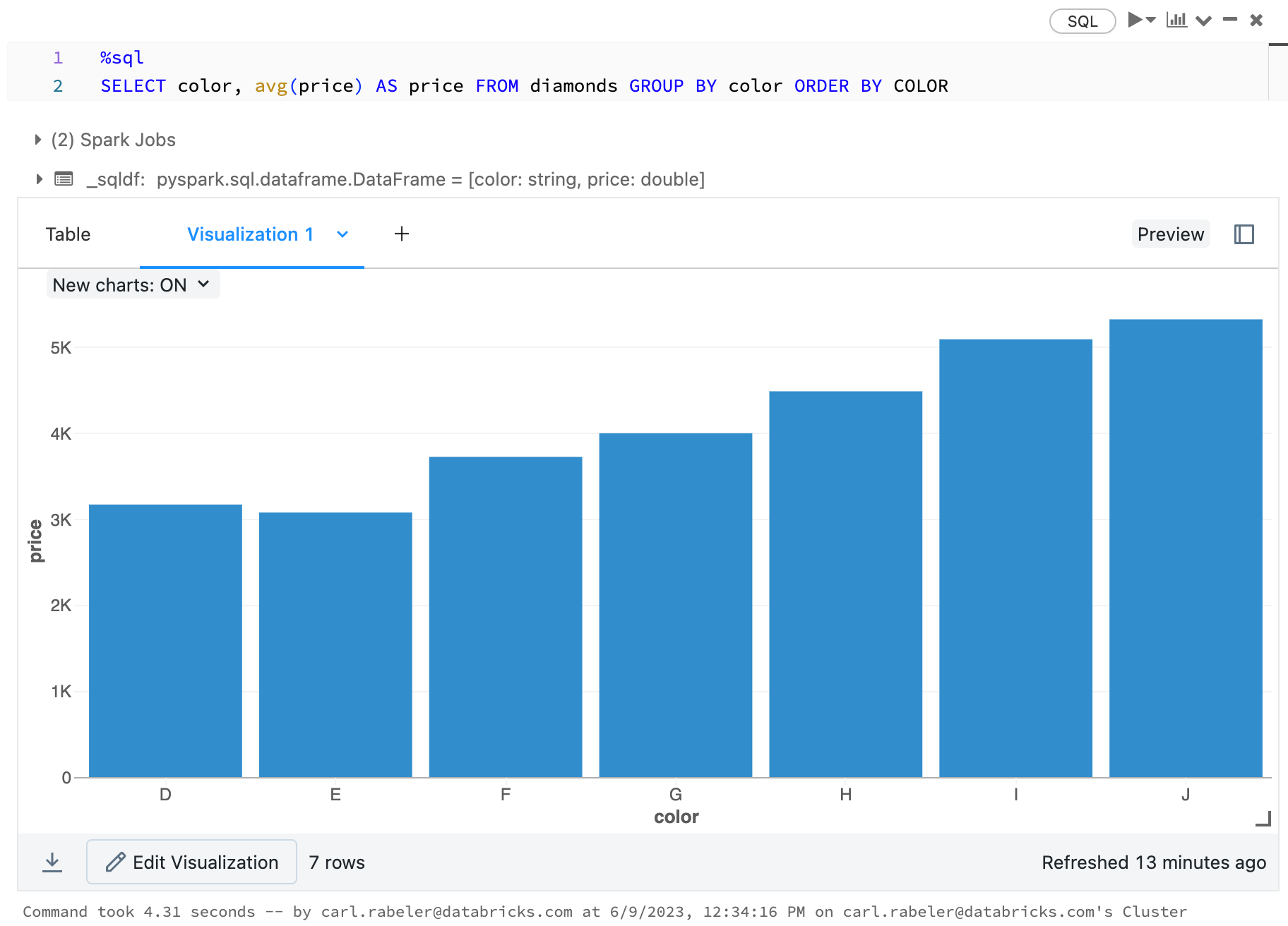You can click the down arrow next to the bar chartto choose another chart type and click Plot Options... to configure the chart.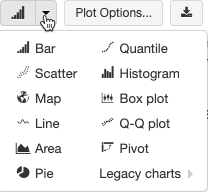#### Series coloring

Databricks supports two kinds of color consistency across charts: series set and global.

Series set color consistency assigns the same color to the same value if you have series with the same values but in different orders (for example, A = `["Apple", "Orange", "Banana"]` and B = `["Orange", "Banana", "Apple"]`). The values are sorted before plotting, so both legends are sorted the same way (`["Apple", "Banana", "Orange"]`), and the same values are given the same colors. However, if you have a series C = `["Orange", "Banana"]`, it would not be color consistent with set A because the set isn’t the same. The sorting algorithm would assign the first color to “Banana” in set C but the second color to “Banana” in set A. If you want these series to be color consistent, you can specify that charts should have global color consistency.

In global color consistency, each value is always mapped to the same color no matter what values the series have. To enable this for each chart, select the Global color consistency checkbox.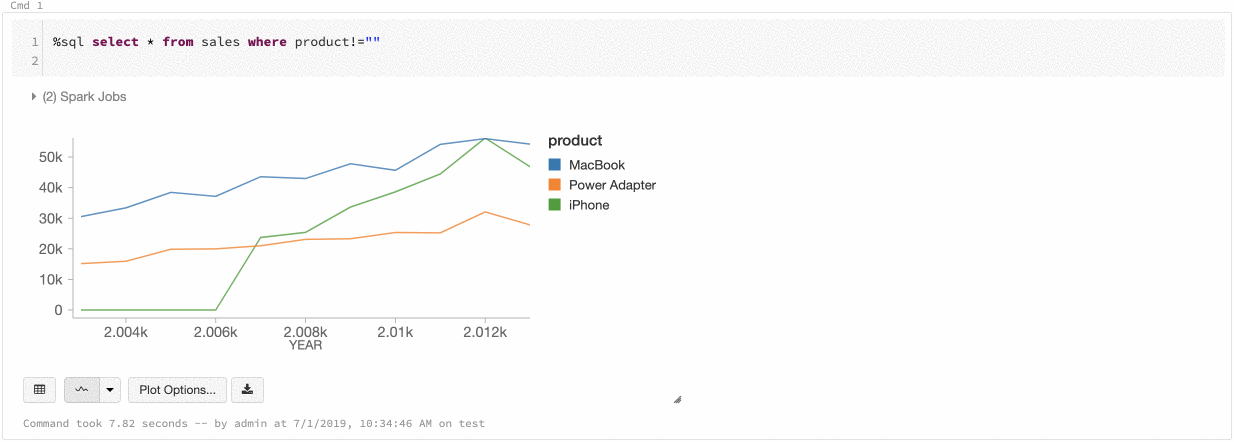Note

To do achieve this consistency, Databricks hashes directly from values to colors. To avoid collisions (where two values go to the exact same color), the hash is to a large set of colors, which has the side effect that nice-looking or easily distinguishable colors cannot be guaranteed; with many colors there are bound to be some that are very similar looking.

### Images types in DataFrames

`display` renders columns containing image data types as rich HTML. For clusters running Databricks Runtime 4.1 and above, `display` attempts to render image thumbnails for `DataFrame` columns matching Spark’s ImageSchema. Thumbnail rendering works for any images successfully read in through the readImages function. For image values generated through other means, Databricks supports the rendering of 1, 3, or 4 channel images (where each channel consists of a single byte), with the following constraints:

• One-channel images: `mode` field must be equal to 0. `height`, `width`, and `nChannels` fields must accurately describe the binary image data in the `data` field
• Three-channel images: `mode` field must be equal to 16. `height`, `width`, and `nChannels` fields must accurately describe the binary image data in the `data` field. The `data` field must contain pixel data in three-byte chunks, with the channel ordering `(blue, green, red)` for each pixel.
• Four-channel images: `mode` field must be equal to 24. `height`, `width`, and `nChannels` fields must accurately describe the binary image data in the `data` field. The `data` field must contain pixel data in four-byte chunks, with the channel ordering `(blue, green, red, alpha)` for each pixel.

Example

Suppose you have a folder containing some images: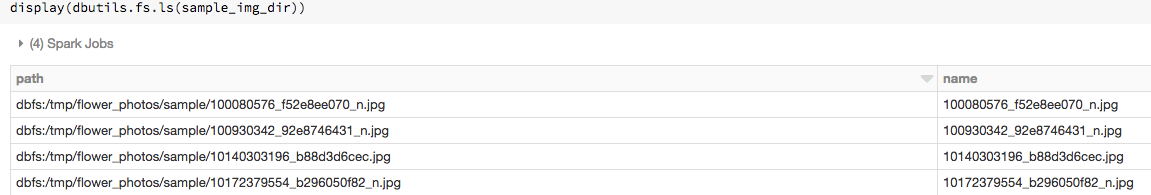If you read the images into a DataFrame with `ImageSchema.readImages` and then display the DataFrame, `display` renders thumbnails of the images:

```from pyspark.ml.image import ImageSchema
display(image_df)
```### Machine learning visualizations

The `display` function supports various machine learning algorithm visualizations.

In this section:

#### Residuals

For linear and logistic regressions, `display` supports rendering a fitted versus residuals plot. To obtain this plot, you supply the model and DataFrame.

The following example runs a linear regression on city population to house sale price data and then displays the residuals versus the fitted data.

```# Load data

# Drop rows with missing values and rename the feature and label columns, replacing spaces with _
pop_df = data.dropna() # drop rows with missing values
exprs = [col(column).alias(column.replace(' ', '_')) for column in data.columns]

# Register a UDF to convert the feature (2014_Population_estimate) column vector to a VectorUDT type and apply it to the column.
from pyspark.ml.linalg import Vectors, VectorUDT

spark.udf.register("oneElementVec", lambda d: Vectors.dense([d]), returnType=VectorUDT())
tdata = data.select(*exprs).selectExpr("oneElementVec(2014_Population_estimate) as features", "2015_median_sales_price as label")

# Run a linear regression
from pyspark.ml.regression import LinearRegression

lr = LinearRegression()
modelA = lr.fit(tdata, {lr.regParam:0.0})

# Plot residuals versus fitted data
display(modelA, tdata)
```

#### ROC curves

For logistic regressions, `display` supports rendering an ROC curve. To obtain this plot, you supply the model, the prepped data that is input to the `fit` method, and the parameter `"ROC"`.

The following example develops a classifier that predicts if an individual earns <=50K or >50k a year from various attributes of the individual. The Adult dataset derives from census data, and consists of information about 48842 individuals and their annual income.

```CREATE TABLE adult (
age DOUBLE,
workclass STRING,
fnlwgt DOUBLE,
education STRING,
education_num DOUBLE,
marital_status STRING,
occupation STRING,
relationship STRING,
race STRING,
sex STRING,
capital_gain DOUBLE,
capital_loss DOUBLE,
hours_per_week DOUBLE,
native_country STRING,
income STRING)
USING CSV
```
```dataset = spark.table("adult")

# Use One-Hot Encoding to convert all categorical variables into binary vectors.

from pyspark.ml import Pipeline
from pyspark.ml.feature import OneHotEncoderEstimator, StringIndexer, VectorAssembler
categoricalColumns = ["workclass", "education", "marital_status", "occupation", "relationship", "race", "sex", "native_country"]

stages = [] # stages in our Pipeline
for categoricalCol in categoricalColumns:
# Category Indexing with StringIndexer
stringIndexer = StringIndexer(inputCol=categoricalCol, outputCol=categoricalCol + "Index")
# Use OneHotEncoder to convert categorical variables into binary SparseVectors
# encoder = OneHotEncoderEstimator(inputCol=categoricalCol + "Index", outputCol=categoricalCol + "classVec")
encoder = OneHotEncoderEstimator(inputCols=[stringIndexer.getOutputCol()], outputCols=[categoricalCol + "classVec"])
# Add stages.  These are not run here, but will run all at once later on.
stages += [stringIndexer, encoder]

# Convert label into label indices using the StringIndexer
label_stringIdx = StringIndexer(inputCol="income", outputCol="label")
stages += [label_stringIdx]

# Transform all features into a vector using VectorAssembler
numericCols = ["age", "fnlwgt", "education_num", "capital_gain", "capital_loss", "hours_per_week"]
assemblerInputs = [c + "classVec" for c in categoricalColumns] + numericCols
assembler = VectorAssembler(inputCols=assemblerInputs, outputCol="features")
stages += [assembler]

# Run the stages as a Pipeline. This puts the data through all of the feature transformations in a single call.

partialPipeline = Pipeline().setStages(stages)
pipelineModel = partialPipeline.fit(dataset)

# Fit logistic regression model

from pyspark.ml.classification import LogisticRegression

# ROC for data
```

To display the residuals, omit the `"ROC"` parameter:

```display(lrModel, preppedDataDF)
```

#### Decision trees

The `display` function supports rendering a decision tree. This is supported for Scala in Databricks Runtime 4.1 and above and for Python in Databricks Runtime 4.3 and above.

To obtain this visualization, you supply the decision tree model.

The following examples train a tree to recognize digits (0 - 9) from the MNIST dataset of images of handwritten digits and then displays the tree.

Scala

```val trainingDF = spark.read.format("libsvm").load("/databricks-datasets/mnist-digits/data-001/mnist-digits-train.txt").cache

import org.apache.spark.ml.classification.{DecisionTreeClassifier, DecisionTreeClassificationModel}
import org.apache.spark.ml.feature.StringIndexer
import org.apache.spark.ml.Pipeline

val indexer = new StringIndexer().setInputCol("label").setOutputCol("indexedLabel")
val dtc = new DecisionTreeClassifier().setLabelCol("indexedLabel")
val pipeline = new Pipeline().setStages(Array(indexer, dtc))

val model = pipeline.fit(trainingDF)
val tree = model.stages.last.asInstanceOf[DecisionTreeClassificationModel]

display(tree)
```

Python

```trainingDF = spark.read.format("libsvm").load("/databricks-datasets/mnist-digits/data-001/mnist-digits-train.txt").cache()

from pyspark.ml.classification import DecisionTreeClassifier
from pyspark.ml.feature import StringIndexer
from pyspark.ml import Pipeline

indexer = StringIndexer().setInputCol("label").setOutputCol("indexedLabel")

dtc = DecisionTreeClassifier().setLabelCol("indexedLabel")

# Chain indexer + dtc together into a single ML Pipeline.
pipeline = Pipeline().setStages([indexer, dtc])

model = pipeline.fit(trainingDF)
display(model.stages[-1])
```

### Visualizations in Python

To create a visualization in Python, call `display(<dataframe-name>)`.

```diamonds_df = spark.read.csv("/databricks-datasets/Rdatasets/data-001/csv/ggplot2/diamonds.csv", header="true", inferSchema="true")

display(diamonds_df.groupBy("color").avg("price").orderBy("color"))
```For a deep dive into Python visualizations using `display`, see Visualization Deep Dive in Python.

For visualizations specific to machine learning, see Machine learning visualizations.

You can also use other Python libraries to generate visualizations. The Databricks Runtime includes the seaborn visualization library so it’s easy to create a seaborn plot. For example:

```import seaborn as sns
sns.set(style="white")

g = sns.PairGrid(df, diag_sharey=False)
g.map_lower(sns.kdeplot)
g.map_diag(sns.kdeplot, lw=3)

g.map_upper(sns.regplot)

display(g.fig)
```

For other libraries and examples, see Matplotlib and ggplot in Python Notebooks, Bokeh in Python Notebooks, and Plotly in Python and R Notebooks.

### Visualizations in R

In addition to the Databricks visualizations, R notebooks can use any R visualization package. The R notebook will capture the resulting plot as a `.png` and display it inline.

`display` function

```library(SparkR)
diamonds_df <- read.df("/databricks-datasets/Rdatasets/data-001/csv/ggplot2/diamonds.csv", source = "csv", header="true", inferSchema = "true")

display(arrange(agg(groupBy(diamonds_df, "color"), "price" = "avg"), "color"))
```

Default library

```fit <- lm(Petal.Length ~., data = iris)
layout(matrix(c(1,2,3,4),2,2)) # optional 4 graphs/page
plot(fit)
```

ggplot

```library(ggplot2)
ggplot(diamonds, aes(carat, price, color = color, group = 1)) + geom_point(alpha = 0.3) + stat_smooth()
```

Lattice

```library(lattice)
xyplot(price ~ carat | cut, diamonds, scales = list(log = TRUE), type = c("p", "g", "smooth"), ylab = "Log price")
```

You can also install and use other plotting libraries.

```install.packages("DandEFA", repos = "http://cran.us.r-project.org")
library(DandEFA)
data(timss2011)
timss2011 <- na.omit(timss2011)
dandpal <- rev(rainbow(100, start = 0, end = 0.2))
dandelion(facl,bound=0,mcex=c(1,1.2),palet=dandpal)
dandelion(facl,bound=0,mcex=c(1,1.2),palet=dandpal)
```

### Visualizations in Scala

The easiest way to perform plotting in Scala is to use the `display` method. For example:

```val diamonds_df = spark.read.format("csv").option("header","true").option("inferSchema","true").load("/databricks-datasets/Rdatasets/data-001/csv/ggplot2/diamonds.csv")

display(diamonds_df.groupBy("color").avg("price").orderBy("color"))
```For a deep dive into Scala visualizations using `display`, see Visualization Deep Dive in Scala.

For visualizations specific to machine learning, see Machine learning visualizations.

### Visualizations in SQL

When you execute SQL, Databricks automatically extracts some of the data and displays it as a table.

```SELECT color, avg(price) AS price FROM diamonds GROUP BY color ORDER BY COLOR
```From there you can select different styles of visualization.## `displayHTML` function

The `displayHTML` iframe is served from the domain `databricksusercontent.com`, and the iframe sandbox includes the `allow-same-origin` attribute. `databricksusercontent.com` must be accessible from your browser. If it is currently blocked by your corporate network, it will need to be whitelisted by IT.

See Embed static images in notebooks and HTML, D3, and SVG in Notebooks for examples of using `displayHTML`.